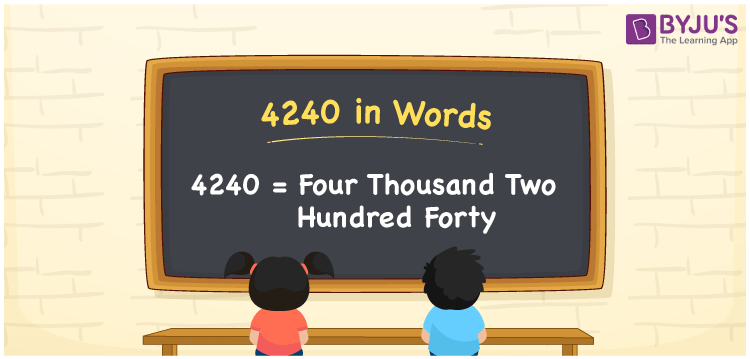# 4240 in words

4240 in words is written as Four Thousand Two Hundred and Forty. In 4240, 4 has a place value of thousand, 2 is in the place value of hundred and next 4 is in the place value of ten. The article on Place Value gives more information. The number 4240 is used in expressions that relate to money, distance, length, area and many more. For example, “That plot measures 80 x 53, which means the area of the plot is Four Thousand Two Hundred and Forty square meters.”

 4240 in words Four Thousand Two Hundred and Forty Four Thousand Two Hundred and Forty in Numbers 4240

## 4240 in English Words## How to Write 4240 in Words?

We can convert 4240 to words using a place value chart. The number 4240 has 4 digits, so let’s make a chart that shows the place value up to 4 digits.

 Thousands Hundreds Tens Ones 4 2 4 0

Thus, we can write the expanded form as:

4 × Thousand + 2 × Hundred + 4 × Ten + 0 × One

= 4 × 1000 + 2 × 100 + 4 × 10 + 0 × 1

= 4240.

= Four Thousand Two Hundred and Forty.

4240 is the natural number that is succeeded by 4239 and preceded by 4241.

4240 in words – Four Thousand Two Hundred and Forty.

Is 4240 an odd number? – No.

Is 4240 an even number? – Yes.

Is 4240 a perfect square number? – No.

Is 4240 a perfect cube number? – No.

Is 4240 a prime number? – No.

Is 4240 a composite number? – Yes.

## Solved Example

1. Write the number 4240 in expanded form

Solution: 4 x 1000 + 2 x 100 + 4 x 10 + 0 x 1

Or Just 4 x 1000 + 2 x 100 + 4 x 10

We can write 4240 = 4000 + 200 + 40 + 0

= 4 x 1000 + 2 x 100 + 4 x 10 + 0 x 1.

## Frequently Asked Questions on 4240 in words

Q1

### How to write the number 4240 in words?

4240 in words is written as Four Thousand Two Hundred and Forty.
Q2

### Is 4240 divisible by 3?

No. 4240 is not divisible by 3.
Q3

### Is 4240 divisible by 10?

Yes. 4240 is divisible by 10.